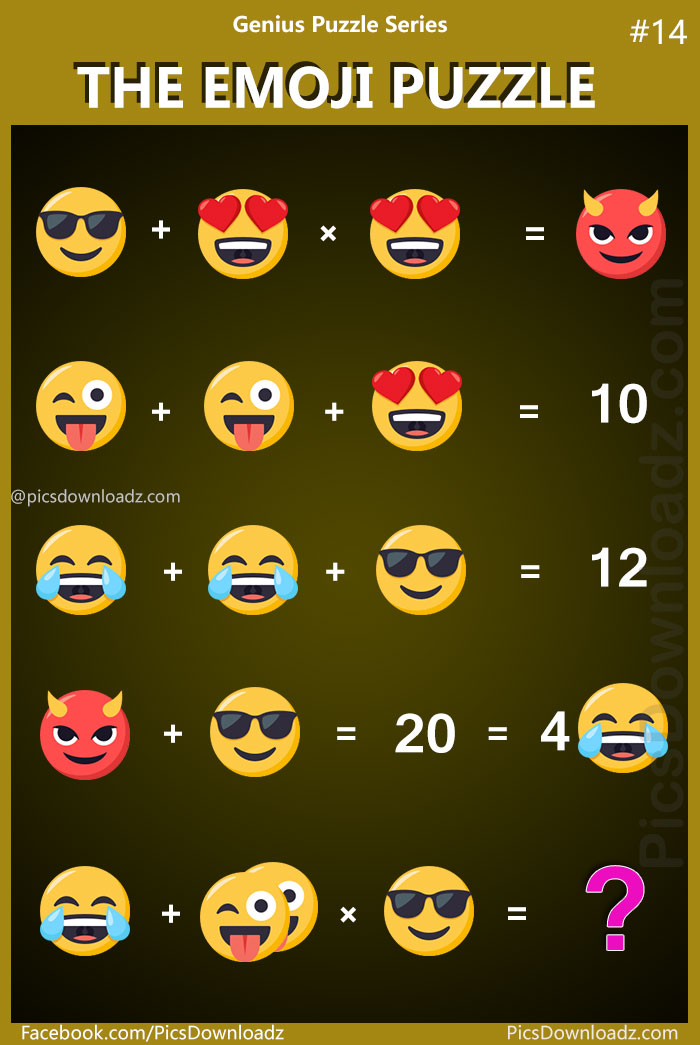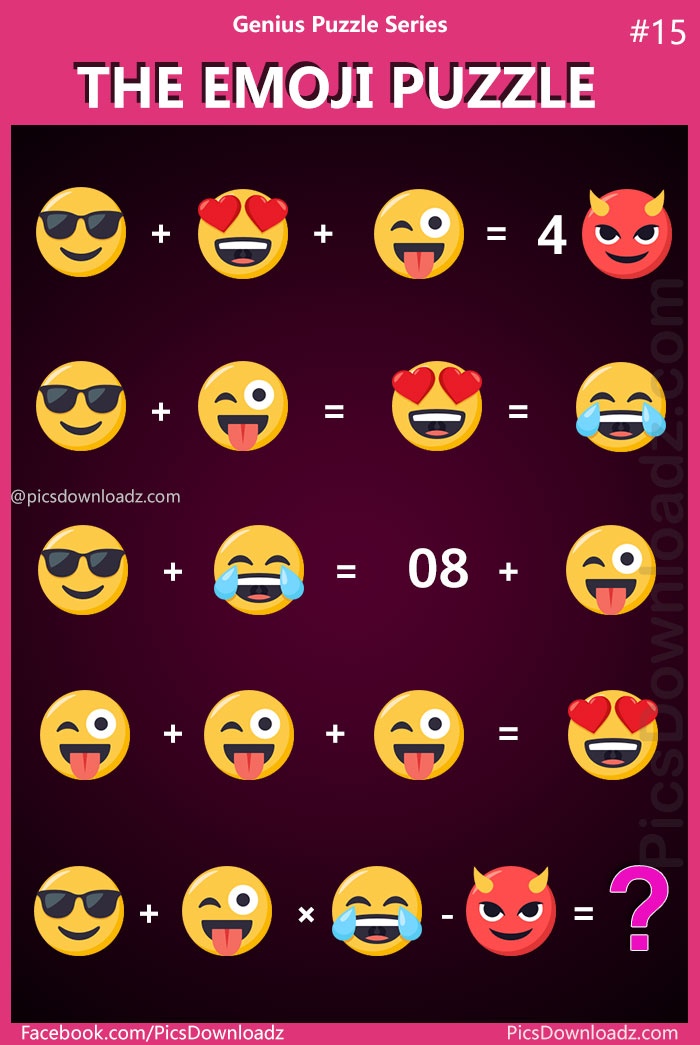This post may contain affiliate links. This means if you click on the link and purchase the item, We will receive an affiliate commission at no extra cost to you. See Our Affiliate Policy for more info.

# The Emoji Puzzle: Genius Puzzle Series #14 – #15 – Viral Facebook Puzzle

2
18574

### Confusing Math Puzzle problem! 90% will fail to attempt this logical brain teaser math puzzle. “The Emoji Puzzle” Most interesting and confusing logic puzzle! Find the correct answer

Whatsup guys!  🙂

Who love Emojis? What a stupid question  😈 of course we all do. Here’s Emoji day special challenge for you.

Welcome back to Genius Puzzle Series, Puzzle #14 & #15. Another interesting brain-teaser logical puzzle for you. Ready? Play with facebook emoji and find out the correct answer.

The Puzzle divided into two parts. The easy one, and the hard level.

Let’s go to the puzzle without wasting your time.

### The Emoji Puzzle: Genius Puzzle Series #14: Level Easy### The Emoji Puzzle: Genius Puzzle Series #15: Level HardLike our Page:

### The Emoji Puzzle Answer #14

#### Solution for #14:

Let’s assume the puzzle icon or equations as follow:

1eq. ⇒ A + B × B = C

2eq. ⇒ D + D + B = 10

3eq. ⇒ E + E + A = 12

4eq. ⇒ C + A = 20 = 4(E)

5eq. ⇒ E + (D+D) × A = ??

#### In 4eq. =>

4E = 20, E = 20/4 = 5, E = 5

#### In 3eq. =>

E + E + A = 12, (put E value from 4eq.), We get A = 2

#### In 4eq. =>

C + A = 20, (put A value from 3eq.), We get C = 18

#### In 1eq. =>

A + B × B = C, (put A & C value from 3eq. and 4eq.), We get B = 4

#### In 2eq. =>

D + D + B = 10, (put B value from 1eq.), We get D = 3

#### Last OR 5eq. =>

E + (D+D) × A = ??

5 + (3+3) × 2  =  5 + 6 × 2  =  17 Ans.

### The Emoji Puzzle Answer #15

Solution: Let’s assume the puzzle icon or equations as follow:

1eq. ⇒ A + B + C = 4D

2eq. ⇒ A + C = B = E

3eq. ⇒ A + E = 8 + C

4eq. ⇒ C + C + C = B

5eq. ⇒ A + C × E – D = ??

#### In 4eq. =>

⇒ 3C = B = E   (B=E from 2eq.)

#### In 3eq. =>

⇒ A + E = 8 + C  (We know E = 3C from 4th eq.)

⇒ A + 3C = 8 + C

⇒ A + 3C – C = 8

⇒ A + 2C = 8

#### In 2eq. =>

⇒ A + C = B   (We know B = 3C from 4th eq.)

⇒ A + C = 3C

⇒ A – 2C = 0

Now Compare latest 2nd & 3rd eq. with each other, you will get the value of A and C.

⇒ A + 2C = 8 (from 3rd eq.)   Vs

⇒ A – 2C = 0 (from 2rd eq.)

A = 4 , C = 2

⇒ A + C = B = E

⇒ 4 + 2 = 6

B = E = 6

#### In 1eq. =>

⇒ A + B + C = 4D  (put their values)

⇒ 4 + 6 + 2 = 4D

⇒ 12 = 4D

⇒ D = 12/4 = 3

D = 3

#### Last OR 5th Eq. =>

⇒ A + C × E – D = ??  (We know A = 4 , C = 2, B = E = 6, D = 3)

⇒ 4 + 2 × 6 – 3

⇒ 4 + 12 – 3

13 Ans.

### Want to print or share on your blog?

Simply download this puzzle image and print it out. Share this puzzle with your kids, students, and fellows. And for blog and website, you are free to use this image but you’re not allowed to edit the image and must attribute the image by linking to the original source, so we will be able to continue creating new puzzles & other amazing posts for you. Love <3

Search items:

Difficult Math Puzzles, Fun maths puzzle questions with answers, logic puzzles for adults, The Emoji Puzzle, Genius Puzzle Series #14, Genius Puzzle Series #15, logic puzzles with answers, Facebook Emoji Puzzle, Puzzle for Facebook, Viral Whatsapp Puzzle, Free Printable Puzzle Image for Competition, Puzzle for School Kids, Printable Math Puzzle for School, Tough Math Puzzle Question, Confusing Math Puzzle Images, Brainteasers Math Puzzles, Only for Genius Math Puzzle, Emoji maths puzzles with solutions, brain math puzzles for adults, brain teasers for adults.

1.K
2.Abdullah Razzu